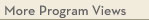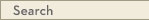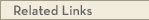# University Catalogs

Twin Cities campusTwin Cities Campus

# Econometrics Minor

Economics
College of Liberal Arts
• Program Type: Undergraduate minor related to major
• Requirements for this program are current for Fall 2020
• Required credits in this minor: 46 to 48
The Econometrics minor appeals to students who plan to pursue careers related to Mathematics, Statistics, and Data Science. The minor will give students the ability to work with economic data, use estimation techniques to prove or disprove their hypothesis, and draw conclusions. Data analysis is a highly marketable skill.
Program Delivery
This program is available:
• via classroom (the majority of instruction is face-to-face)
Required prerequisites
Principles of Micro and Macroeconomics
Take exactly 2 course(s) totaling exactly 8 credit(s) from the following:
· ECON 1101 - Principles of Microeconomics [SOCS, GP] (4.0 cr)
· ECON 1102 - Principles of Macroeconomics (4.0 cr)
Required prerequisites
Calculus I
Take exactly 1 course(s) totaling exactly 4 credit(s) from the following:
· MATH 1271 - Calculus I [MATH] (4.0 cr)
or MATH 1371 - CSE Calculus I [MATH] (4.0 cr)
or MATH 1571H - Honors Calculus I [MATH] (4.0 cr)
Minor Requirements
Students may receive no more than one undergraduate degree from the Department of Economics: a BA or a BS or a minor.
Core Course
Take exactly 1 course(s) totaling exactly 4 credit(s) from the following:
· ECON 3101 - Intermediate Microeconomics (4.0 cr)
Mathematics
Take exactly 3 course(s) totaling exactly 12 credit(s) from the following:
Calculus II
· MATH 1272 - Calculus II (4.0 cr)
or MATH 1372 - CSE Calculus II (4.0 cr)
or MATH 1572H - Honors Calculus II (4.0 cr)
· Linear Algebra and Differential Equations
· MATH 2243 - Linear Algebra and Differential Equations (4.0 cr)
or MATH 2373 - CSE Linear Algebra and Differential Equations (4.0 cr)
or MATH 2574H - Honors Calculus IV (4.0 cr)
· Multivariable Calculus
· MATH 2263 - Multivariable Calculus (4.0 cr)
or MATH 2374 - CSE Multivariable Calculus and Vector Analysis (4.0 cr)
Statistics
Take exactly 2 course(s) totaling exactly 8 credit(s) from the following:
Option 1
· STAT 4101 - Theory of Statistics I (4.0 cr)
STAT 4102 - Theory of Statistics II (4.0 cr)
· Option 2
STAT 5101 - Theory of Statistics I (4.0 cr)
or MATH 5651 - Basic Theory of Probability and Statistics (4.0 cr)
STAT 5102 - Theory of Statistics II (4.0 cr)
or MATH 5652 - Introduction to Stochastic Processes (4.0 cr)
Econometrics
Take exactly 1 course(s) totaling exactly 4 credit(s) from the following:
· ECON 4261 - Introduction to Econometrics (4.0 cr)
Electives
Take exactly 2 course(s) totaling 6 - 8 credit(s) from the following:
· ECON 4115 - Uncertainty and Information (4.0 cr)
· ECON 4118 - Advanced Mathematical Economics (4.0 cr)
· ECON 4631 - Industrial Organization and Antitrust Policy (3.0 cr)
· ECON 4751 - Financial Economics (3.0 cr)
· ECON 4831 - Cost-Benefit Analysis (3.0 cr)View college catalog(s): · College of Liberal Arts View future requirement(s): · Fall 2022 View checkpoint chart: · Econometrics Minor View PDF Version:Search Programs Search University CatalogsOne Stop for tuition, course registration, financial aid, academic calendars, and moreECON 1101 - Principles of Microeconomics (SOCS, GP)
 Credits: 4.0 [max 4.0] Course Equivalencies: Econ 1101/1104/1111/ApEc 1101 Typically offered: Every Fall, Spring & Summer
Microeconomic behavior of consumers, firms, and markets in domestic and world economy. Demand and supply. Competition and monopoly. Distribution of income. Economic interdependencies in the global economy. Effects of global linkages on individual decisions. prereq: knowledge of plane geometry and advanced algebra
ECON 1102 - Principles of Macroeconomics
 Credits: 4.0 [max 4.0] Course Equivalencies: ApEc 1102/Econ 1102/1105/1112 Typically offered: Every Fall, Spring & Summer
Aggregate consumption, saving, investment, and national income. Role of money, banking, and business cycles in domestic and world economy. International trade, growth, and development. U.S. economy and its role in the world economy. International interdependencies among nations. prereq: [1101 or equiv], knowledge of plane geometry and advanced algebra
MATH 1271 - Calculus I (MATH)
 Credits: 4.0 [max 4.0] Course Equivalencies: Math 1271/Math 1281/Math 1371/ Typically offered: Every Fall, Spring & Summer
Differential calculus of functions of a single variable, including polynomial, rational, exponential, and trig functions. Applications, including optimization and related rates problems. Single variable integral calculus, using anti-derivatives and simple substitution. Applications may include area, volume, work problems. prereq: 4 yrs high school math including trig or satisfactory score on placement test or grade of at least C- in [1151 or 1155]
MATH 1371 - CSE Calculus I (MATH)
 Credits: 4.0 [max 4.0] Course Equivalencies: Math 1271/Math 1281/Math 1371/ Typically offered: Every Fall & Spring
Differentiation of single-variable functions, basics of integration of single-variable functions. Applications: max-min, related rates, area, curve-sketching. Use of calculator, cooperative learning. prereq: CSE or pre-bioprod concurrent registration is required (or allowed) in biosys engn (PRE), background in [precalculus, geometry, visualization of functions/graphs], instr consent; familiarity with graphing calculators recommended
MATH 1571H - Honors Calculus I (MATH)
 Credits: 4.0 [max 4.0] Course Equivalencies: Math 1271/Math 1281/Math 1371/ Grading Basis: A-F only Typically offered: Every Fall
Differential/integral calculus of functions of a single variable. Emphasizes hard problem-solving rather than theory. prereq: Honors student and permission of University Honors Program
ECON 3101 - Intermediate Microeconomics
 Credits: 4.0 [max 4.0] Course Equivalencies: Econ 3101/Econ 3101H/ApEc 3001 Grading Basis: A-F only Typically offered: Every Fall, Spring & Summer
Behavior of households, firms, and industries under competitive/monopolistic conditions. Factors influencing production, price, and other decisions. Applications of theory. Economic efficiency. Distribution of well-being. prereq: [[1101, 1102] or equiv], [MATH 1271 or equiv]
MATH 1272 - Calculus II
 Credits: 4.0 [max 4.0] Course Equivalencies: Math 1272/Math 1282/Math 1372/ Typically offered: Every Fall, Spring & Summer
Techniques of integration. Calculus involving transcendental functions, polar coordinates. Taylor polynomials, vectors/curves in space, cylindrical/spherical coordinates. prereq: [1271 or equiv] with grade of at least C-
MATH 1372 - CSE Calculus II
 Credits: 4.0 [max 4.0] Course Equivalencies: Math 1272/Math 1282/Math 1372/ Typically offered: Every Spring
Techniques of integration. Calculus involving transcendental functions, polar coordinates, Taylor polynomials, vectors/curves in space, cylindrical/spherical coordinates. Use of calculators, cooperative learning. prereq: Grade of at least C- in [1371 or equiv], CSE or pre-Bioprod/Biosys Engr
MATH 1572H - Honors Calculus II
 Credits: 4.0 [max 4.0] Course Equivalencies: Math 1272/Math 1282/Math 1372/ Grading Basis: A-F only Typically offered: Every Spring
Continuation of 1571. Infinite series, differential calculus of several variables, introduction to linear algebra. prereq: 1571H, honors student, permission of University Honors Program
MATH 2243 - Linear Algebra and Differential Equations
 Credits: 4.0 [max 4.0] Course Equivalencies: Math 2243/Math 2373/Math 2574H Typically offered: Every Fall, Spring & Summer
Linear algebra: basis, dimension, matrices, eigenvalues/eigenvectors. Differential equations: first-order linear, separable; second-order linear with constant coefficients; linear systems with constant coefficients. prereq: [1272 or 1282 or 1372 or 1572] w/grade of at least C-
MATH 2373 - CSE Linear Algebra and Differential Equations
 Credits: 4.0 [max 4.0] Course Equivalencies: Math 2243/Math 2373/Math 2574H Typically offered: Every Fall & Spring
Linear algebra: basis, dimension, eigenvalues/eigenvectors. Differential equations: linear equations/systems, phase space, forcing/resonance, qualitative/numerical analysis of nonlinear systems, Laplace transforms. Use of computer technology. prereq: [1272 or 1282 or 1372 or 1572] w/grade of at least C-, CSE or pre-Bio Prod/Biosys Engr
MATH 2574H - Honors Calculus IV
 Credits: 4.0 [max 4.0] Course Equivalencies: Math 2243/Math 2373/Math 2574H Grading Basis: A-F only Typically offered: Every Spring
Advanced linear algebra, differential equations. Additional topics as time permits. prereq: Math 1572H or Math 2573H, honors student and permission of University Honors Program
MATH 2263 - Multivariable Calculus
 Credits: 4.0 [max 4.0] Course Equivalencies: Math 2263/Math 2374/Math 2573H Typically offered: Every Fall, Spring & Summer
Derivative as linear map. Differential/integral calculus of functions of several variables, including change of coordinates using Jacobians. Line/surface integrals. Gauss, Green, Stokes Theorems. prereq: [1272 or 1372 or 1572] w/grade of at least C-
MATH 2374 - CSE Multivariable Calculus and Vector Analysis
 Credits: 4.0 [max 4.0] Course Equivalencies: Math 2263/Math 2374/Math 2573H Typically offered: Every Fall & Spring
Derivative as linear map. Differential/integral calculus of functions of several variables, including change of coordinates using Jacobians. Line/surface integrals. Gauss, Green, Stokes theorems. Use of computer technology. prereq: [1272 or 1282 or 1372 or 1572] w/grade of at least C-, CSE or pre-Bioprod/Biosys Engr
STAT 4101 - Theory of Statistics I
 Credits: 4.0 [max 4.0] Typically offered: Every Fall & Spring
Random variables/distributions. Generating functions. Standard distribution families. Data summaries. Sampling distributions. Likelihood/sufficiency. prereq: Math 1272 or Math 1372 or Math 1572H
STAT 4102 - Theory of Statistics II
 Credits: 4.0 [max 4.0] Typically offered: Every Fall & Spring
Estimation. Significance tests. Distribution free methods. Power. Application to regression and to analysis of variance/count data. prereq: STAT 4101
STAT 5101 - Theory of Statistics I
 Credits: 4.0 [max 4.0] Typically offered: Every Fall
Logical development of probability, basic issues in statistics. Probability spaces. Random variables, their distributions and expected values. Law of large numbers, central limit theorem, generating functions, multivariate normal distribution. prereq: (MATH 2263 or MATH 2374 or MATH 2573H), (CSCI 2033 or MATH 2373 or MATH 2243)
MATH 5651 - Basic Theory of Probability and Statistics
 Credits: 4.0 [max 4.0] Course Equivalencies: Math 5651/Stat 5101 Typically offered: Every Fall & Spring
Logical development of probability, basic issues in statistics. Probability spaces, random variables, their distributions/expected values. Law of large numbers, central limit theorem, generating functions, sampling, sufficiency, estimation. prereq: [2263 or 2374 or 2573], [2243 or 2373]; [2283 or 2574 or 3283] recommended.
STAT 5102 - Theory of Statistics II
 Credits: 4.0 [max 4.0] Typically offered: Every Fall & Spring
Sampling, sufficiency, estimation, test of hypotheses, size/power. Categorical data. Contingency tables. Linear models. Decision theory. prereq: [5101 or Math 5651 or instr consent]
MATH 5652 - Introduction to Stochastic Processes
 Credits: 4.0 [max 4.0] Typically offered: Every Fall & Spring
Random walks, Markov chains, branching processes, martingales, queuing theory, Brownian motion. prereq: 5651 or Stat 5101
ECON 4261 - Introduction to Econometrics
 Credits: 4.0 [max 4.0] Grading Basis: A-F or Aud Typically offered: Every Fall, Spring & Summer
For Econ B.S. majors only. Review of basic linear regression model, its variants. Time series/simultaneous equation models. Material may include panel data, censored/truncated regressions, discrete choice models. prereq: [3101 or equiv], [[Math 1271, Math 1272] or equiv], Math 2243, Math 2263, [[Stat 4101, Stat 4102] or [Stat 5101, Stat 5102]]; Math 4242 strongly recommended
ECON 4115 - Uncertainty and Information
 Credits: 4.0 [max 4.0] Grading Basis: A-F only Typically offered: Every Fall & Spring
For Econ B.S. students only. This Microeconomic theory course focuses on economies under uncertainty with possibly asymmetric information. Individual behavior of consumers and that of markets are studied under uncertainty with incomplete information. Related topics in technology and innovation. the information economy, and networks will be examined. prereq: [[3101, 3102]] or equiv], [[MATH 1271, MATH 1272, and Math 2243] or equiv]; strongly suggest students complete Stat 4101 before taking this course; students should have successfully completed two 4xxx level UMNTC economics courses.
ECON 4118 - Advanced Mathematical Economics
 Credits: 4.0 [max 4.0] Course Equivalencies: Econ 4113/Econ 4113H/Econ 4118 Grading Basis: A-F only Typically offered: Periodic Fall & Spring
Development of selected models of economic behavior in mathematical terms. Topics selected to illustrate advantages of mathematical formulation. prereq: ECON 3101, 3102, MATH 1271, MATH 1272, MATH 2243 or equivalent courses; and at least two 4xxx level economics electives.
ECON 4631 - Industrial Organization and Antitrust Policy
 Credits: 3.0 [max 3.0] Course Equivalencies: Econ 3601/Econ 4631/Econ 4631H Typically offered: Every Fall, Spring & Summer
Relations between market structure, economic efficiency and welfare. Economic origins of monopoly and other restraints on competition. Purposes and effects of antitrust and related legislation. Industrial policy. prereq: 3101 or equiv
ECON 4751 - Financial Economics
 Credits: 3.0 [max 3.0] Course Equivalencies: Econ 4751/Econ 4751H/Econ 4758 Grading Basis: A-F only Typically offered: Every Fall, Spring & Summer
Financial decisions of firms/investors. Determination of interest rates and asset prices. Role of risk/uncertainty. Emphasizes economic models. prereq: [3101 or equiv], [MATH 1271 or equiv], one sem statistics
ECON 4831 - Cost-Benefit Analysis
 Credits: 3.0 [max 3.0] Course Equivalencies: Econ 4611H/Econ 4831 Grading Basis: A-F only Typically offered: Every Fall, Spring & Summer
Evaluation of benefits and costs of public projects and programs. Issues connected with definition and measurement of benefits and costs. Rate of return and discount. Market imperfections, risk, uncertainty. Case studies. prereq: ECON 3101